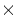Next: Reserved Keywords for Binary Up: Binary Tables Previous: Binary Tables

3.6.1 Required Keywords for Binary Table Extension Headers

The extension follows the rules for required keywords stated in section 3.3.2. The first eight keywords must appear consecutively in the following order:

1.
XTENSION (character) has the value 'BINTABLE' for binary table extensions
2.
BITPIX (integer) must have a value of 8. A binary table is an array of bytes.

3.
NAXIS (integer) must have a value of 2 for binary table extensions. As with ASCII tables, the axes are the rows and columns of the table.

4.
NAXIS1 (integer) has a value equal to the number of 8-bit bytes in a table row.

5.
NAXIS2 (integer) has a value equal to the number of rows in the table. Values of 0 (no table) and 1 (one row), are allowed as well as larger values.

6.
PCOUNT (integer) is equal to the number of bytes in the binary table extension data following the main table. Note that this meaning is significantly different from that in the rules for random groups (section 3.2). This usage was designed for the variable length convention discussed in section 5.2.1. While conflicting uses would not be against the formal FITS rules, they would create confusion and should be avoided. Non-conflicting uses may be developed for other conventions.

7.
GCOUNT (integer) has the value of 1 for binary table extensions.

The total number of bytes is PCOUNT + NAXIS1NAXIS2.

8.
TFIELDS (integer) has a value equal to the number of entries (fields) in each row of the table.

Also required, between the TFIELDS and END keywords, are TFORMn keywords for n=1, ...,TFIELDS.

TFORMn (character) has a value specifying the width and data type of field n and is of the form rT, where T is the field type and r is the repeat count that tells how many values of type T the field contains. The value of r gives the number of members in the array that table item contains. If the repeat count is absent, it is assumed to be 1. A repeat count of zero is permitted. Additional characters may follow the data type code. While these characters are not defined in the formal BINTABLE rules, the variable length array and string array conventions make particular interpretations, and conflicting uses should be avoided, to prevent confusion. The following values of T are permitted:

L
Logical value.
X
Bit array.

A
Character. The substring array convention (section 5.2.2) uses the characters :SSTR immediately following the rA to indicate that the convention is being used to describe the substrings. This usage implies that a colon and different standard character string following the rA can be used as an indicator of other conventions for describing character fields. While the formal FITS rules do not prohibit other uses, such conflicting usage could be confusing and is to be avoided.

B
Unsigned 8-bit integer or byte.

I
Signed 16-bit integer.

J
Signed 32-bit integer.

E
Thirty-two bit IEEE floating point.

D
Sixty-four bit IEEE floating point.

C
Complex pair of single precision floating point values.

M
Complex pair of double precision floating point values.

P
Sixty-four bit descriptor of variable length arrays (pointer). The only allowed repeat counts are 0 and 1. This data type was designed to contain information on the location of data in variable length arrays (section 5.2.1). When used to indicate a variable length array, the value takes the form rPt(maxelem), where r is the repeat count, t is any of the data types discussed here except P, and maxelem is an integer. While the binary table rules do not specifically forbid the use of this data type for other purposes, any use that conflicts with the variable length convention would cause confusion and is to be avoided.

For example, TFORM4  = '16E     ' means that the fourth field of the table will consist of 16 32-bit floating point values. The repeat count represents a major enhancement over ASCII tables, where a field can contain only one value. The number of bytes in a row is the sum of the number of bytes in the individual fields and must equal the value of NAXIS1. Note that this rule differs from ASCII tables, which allow the value of NAXIS1 to be greater than the sum of the TFORMn.

As with ASCII tables, to distinguish -0 from +0 in integer format, for example in degrees of declination, the sign field should be declared as a separate character field. This separation is unecessary for floating point.

END must always be the last keyword. The remainder of the last header record must be filled with ASCII blanks.

Note that while the rules for generalized extensions do not specify where the PCOUNT and GCOUNT keywords are located after the last NAXISn keyword, the rules for binary table extensions require that they appear between the NAXIS2 and TFIELDS keywords.Next: Reserved Keywords for Binary Up: Binary Tables Previous: Binary Tables